5-2 K-nearest-neighbor Classifiers

[chinese][all]

The k-nearest-neighbor classifier (KNNC for short) is one of the most basic classifiers for pattern recognition or data classification. The principle of this method is based on the intuitive concept that data instances of the same class should be closer in the feature space. As a result, for a given data point x of unknown class, we can simply compute the distance between x and all the data points in the training data, and assign the class determined by the K nearest points of x. Due to its simplicity, KNNC is often used as a baseline method in comparison with other sophisticated approaches in pattern recognition.

We shall define KNNC in a more rigorous manner. Suppose that we are given a training dataset of n points with their desired class, as shown below: $$\{(\mathbf{x}_i, y_i), (\mathbf{x}_2, y_2), \dots, (\mathbf{x}_n, y_n)\}$$

where (xi, yi) represent data pair i, with xi as the feature vector and yi as the corresponding target class. Then for a new data point x, the most likely class should be determined by KNNC (k = 1 in this case), as follows: $$nnc(\mathbf{x}, 1) = y_p, p = \arg \min_i \| \mathbf{x} - \mathbf{x}_i \|^2.$$

The preceding equation uses the nearest neighbor to determine the class. Alternatively, we can have K nearest neighbors to determine the class by voting. As the extension to KNNC is straightforward, we shall not formulate it separately.

In the next example, we shall apply KNNC with K=1 for the IRIS dataset, where odd and even indexed entries are used as training and test data, respectively.

Example 1: knnc01.m% Get the data set [trainSet, testSet]=prData('iris'); trainNum=size(trainSet.input, 2); testNum =size(testSet.input, 2); fprintf('Use of KNNC for Iris data:\n'); fprintf('\tSize of design set (odd-indexed data)= %d\n', trainNum); fprintf('\tSize of test set (even-indexed data) = %d\n', testNum); trainSet.k=1; computed=knncEval(testSet, trainSet); correctCount=sum(testSet.output==computed); recogRate=correctCount/testNum; fprintf('Recognition rate = %g%%\n', recogRate*100);Use of KNNC for Iris data: Size of design set (odd-indexed data)= 75 Size of test set (even-indexed data) = 75 Recognition rate = 96%

From the above example, the final recognition rate of KNNC for iris dataset is 96%.

In the 2-dimensional space, the decision boundaries formed by KNNC with k = 1 can be derived from the Voronoi diagram, as explained below:

1. Create the Delauny triangles from the training data points.
2. Identify the perpendicular bisector for each side of the triangle. These perpendicular bisectors form small cells, also known as the Voronoi diagram.
3. Determine the class of a cell according to the class of the data point contained in this cell.
4. Boundaries between cells of different classes are the decision boundaries for 1NNC.
The following example demonstrates the decision boundary formed by 1NNC:

Example 2: knncPlot02.mDS=prData('3classes'); knncPlot(DS, [], 'decBoundary');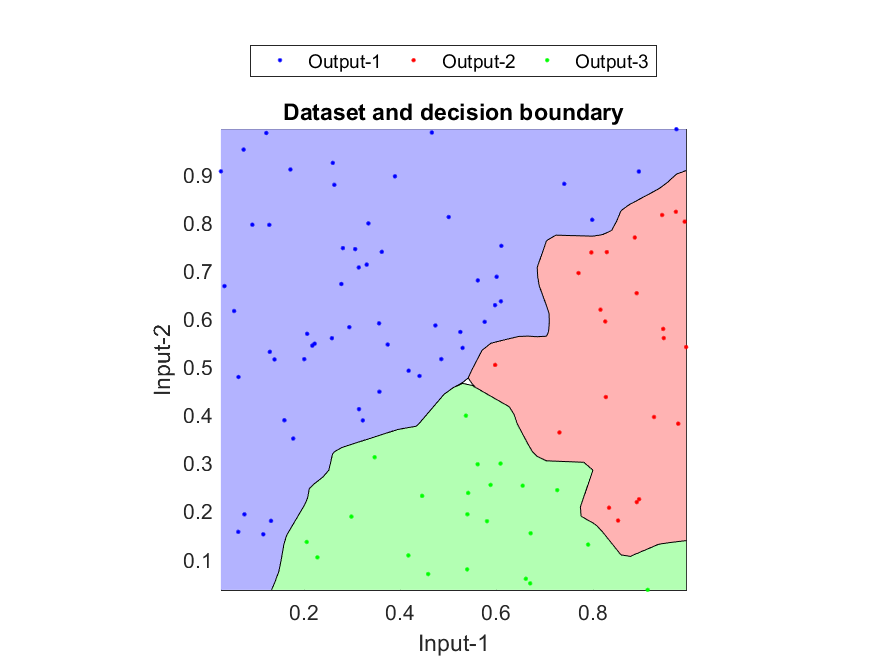We can also compute the distance to each class for a given input domain, and use the distance to create a posterior-like surface for each class:

Example 3: knncPlot01.mDS=prData('3classes'); knncPrm=knncTrain(DS); knncPrm.k=1; knncPlot(DS, knncPrm, '2dPosterior');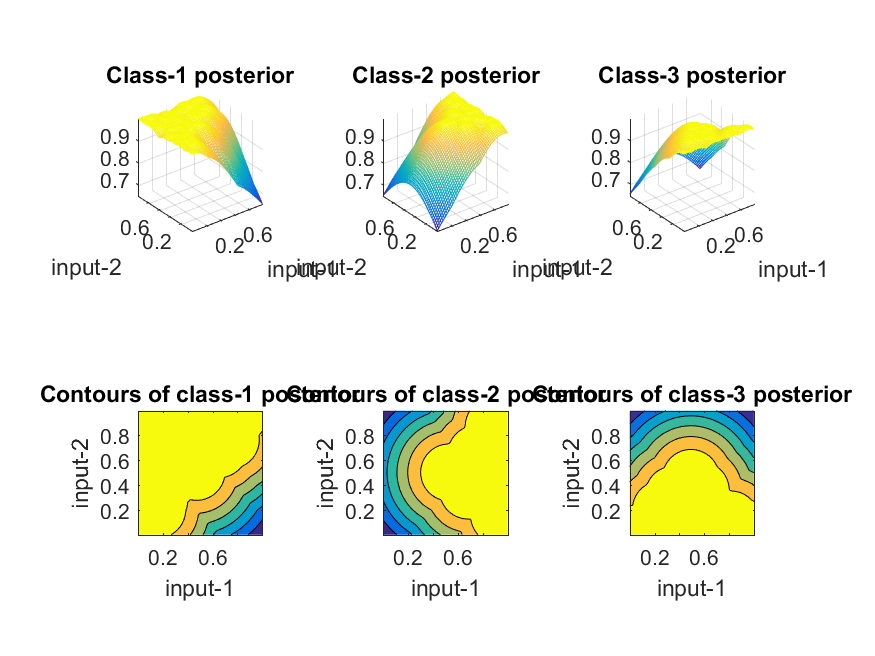Hint
If you want to try an interactive demo of the Voronoi diagram, please change directory to the 'cleve' directory under the example directory of this book and type "vshow" under MATLAB. The demo is by Cleve Moler, to whom the author would like to acknowledge with gratitude.

In the first example, we have computed the recognition rate to be 96% without breakdown performance of each class. If we want to know the performance of each class, we can use the confusion matrix to display the result, as shown in the next example.

Example 4: knnc02.m% Get the data set [trainSet, testSet]=prData('iris'); trainNum=size(trainSet.input, 2); testNum =size(testSet.input, 2); trainSet.k=1; computed=knncEval(testSet, trainSet); confMat=confMatGet(testSet.output, computed); opt=confMatPlot('defaultOpt'); opt.className=trainSet.outputName; confMatPlot(confMat, opt);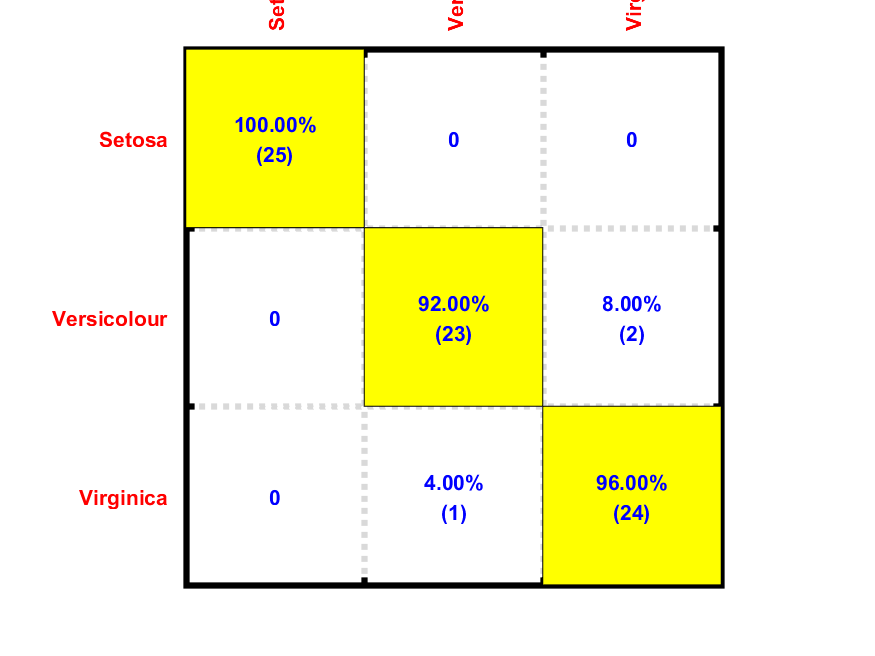In the above example, the left plot is the confusion matrix C where C(i, j) is the number of data in class i being classified as class j. The right plot is the corresponding recognition rates for each class. From the confusion matrix, the recognition rates for classes 1, 2, and 3 are 100%, 96%, and 92%, respectively, with an overall recognition rate of 96%. Moreover, from the confusion matrix, we know that classes 2 and 3 are more likely to be confused since there are only 3 cases of misclassification, one is 2 => 3, the other 3 => 2. (Here we use "i => j" to indicate an entry in class i being classified as class j.)

If the training data is well separated among different classes, then KNNC can usually achieve a satisfactory recognition rate. However, the performance (in terms of computing time and recognition rates) of KNNC degrades in the following situations:

• When the size of the training data is large, then the computation of KNNC is time consuming. This can be a result of either a large size of the training dataset, or a large dimension of the feature vectors.
• The performance of KNNC can also degrade as a result of noisy training data. In this case, we need to trim the training data or simply revert to other recognition methods to avoid the influence by noise.

An alternative to alleviate the above problems is to employ k-means (or the like) to select representative points of each class for KNNC. This procedure can effectively reduce KNNC computation, with a side benefit of removing the effect of noisy training data. Using k-means to help classification involves two steps:

1. For each class, use k-means (or the like) to select representative points for each class. The number of representative points should be much smaller than the original data points in a class.
2. Use the representative points for KNNC.
In the following example, we use k-means to reduce the IRIS dataset for KNNC, where the training data size is reduced from 25 to 3 for each class, as shown next.

Example 5: knncViaKmeans01.m% ====== Get the data set [trainSet, testSet]=prData('iris'); % Get the data set fprintf('Use of KNNC for Iris data:\n'); fprintf('\tSize of design set (odd-indexed data)= %d\n', size(trainSet.input, 2)); fprintf('\tSize of test set (even-indexed data) = %d\n', size(testSet.input, 2)); uniqueClass=unique(trainSet.output); centerNum4eachClass=4; % ====== Perform k-means trainSet2=trainSet; % trainSet2 is the reduced design set after k-means trainSet2.input=[]; trainSet2.output=[]; for i=1:length(uniqueClass) % Compute trainSet2 using k-means index=find(trainSet.output==uniqueClass(i)); thisData=trainSet.input(:, index); center = kMeansClustering(thisData, centerNum4eachClass); trainSet2.input=[trainSet2.input, center]; trainSet2.output=[trainSet2.output, uniqueClass(i)*ones(1, centerNum4eachClass)]; end fprintf('After k-means clustering, size of design set = %d\n', size(trainSet2.input, 2)); % ====== Compute the recognition rate trainSet2.k=1; computed=knncEval(testSet, trainSet2); correctCount=sum(testSet.output==computed); recogRate=correctCount/length(testSet.output); fprintf('Recognition rate = %g%%\n', recogRate*100); % ====== Plot the confusion matrix confMat=confMatGet(testSet.output, computed); opt=confMatPlot('defaultOpt'); opt.className=trainSet.outputName; confMatPlot(confMat, opt);Use of KNNC for Iris data: Size of design set (odd-indexed data)= 75 Size of test set (even-indexed data) = 75 After k-means clustering, size of design set = 12 Recognition rate = 97.3333%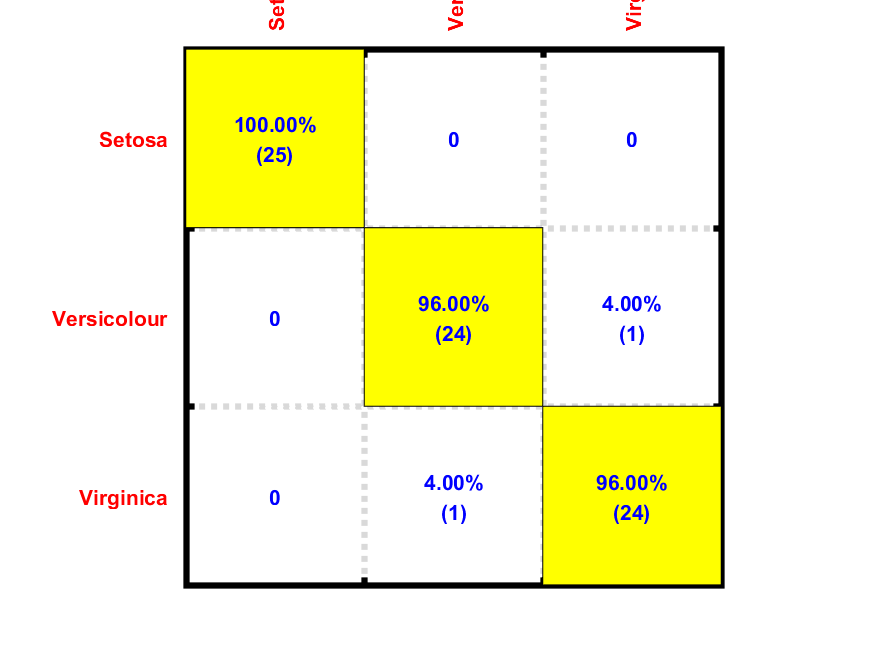Though the size of training data was reduced from 25 to 4 per class, the overall recognition rate is increased from 96% to 98.67%. (Two less cases of 3 => 2). This example demonstrates that data reduction using k-means not only reduce the data size but also increase the recognition rate. However, in general, we do not know the best number of representative points for each class in advance. One way to identify the best number of representative points is to resort to exhaustive search, which is left as an exercise. Since the use of k-means clustering for generating prototypes for each class is commonly adopted in KNNC, we have put the mechanism into a function knncTrain.m. Example follows:

Example 6: knncPlot04.m[trainSet, testSet]=prData('3classes'); knncTrainPrm.method='kMeans'; knncTrainPrm.centerNum4eachClass=4; knncPrm=knncTrain(trainSet, knncTrainPrm); knncPrm.k=1; computed=knncEval(testSet, knncPrm); testSet.hitIndex=find(computed==testSet.output); knncPlot(testSet, knncPrm, 'decBoundary');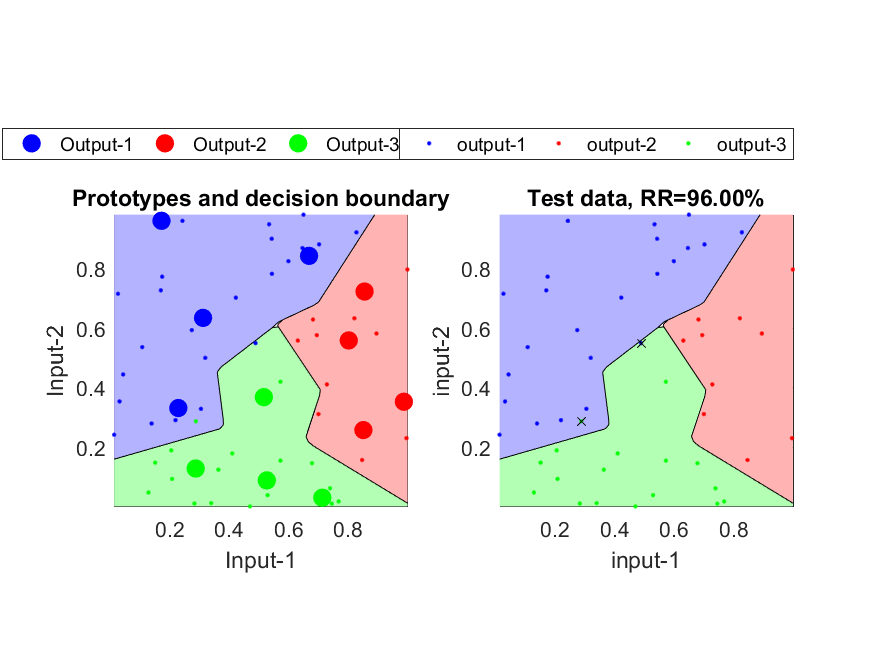It should be noted that the k-means based data reduction is performed on each class sequentially. It only takes intra-class data distribution into consideration. If we want to take inter-class data distribution into consideration for better performance, we can apply LVQ (learning vector quantization) after k-means subsequently. LVQ will be covered in the next section.

If there is a lot of overlap between any two classes in the dataset, it would be more robust to use KNNC with k larger than 1, where the final decision is based on the voting results among these k nearest neighbors. Since there are k data points around the neighborhood to join the decision process, the result is usually more robust. In the following example, we apply KNNC to the IRIS dataset and let the value of K varies from 1 to 15 to see the corresponding recognition rates, as follows.

Example 7: knncRecogVsK01.m% Get the data set [trainSet, testSet]=prData('iris'); designNum=size(trainSet.input, 2); testNum =size(testSet.input, 2); fprintf('Use of KNNC for Iris data:\n'); fprintf('\tSize of design set (odd-indexed data)= %d\n', designNum); fprintf('\tSize of test set (even-indexed data) = %d\n', testNum); fprintf('\tRecognition rates as K varies:\n'); kMax=15; for k=1:kMax trainSet.k=k; computed=knncEval(testSet, trainSet); correctCount=sum(testSet.output==computed); recog(k)=correctCount/testNum; fprintf('\t%d-NNC ===> RR = 1-%d/%d = %.2f%%.\n', k, testNum-correctCount, testNum, recog(k)*100); end plot(1:kMax, recog*100, 'b-o'); grid on; title('Recognition rates of Iris data using K-NNR'); xlabel('K'); ylabel('Recognition rates (%)');Use of KNNC for Iris data: Size of design set (odd-indexed data)= 75 Size of test set (even-indexed data) = 75 Recognition rates as K varies: 1-NNC ===> RR = 1-3/75 = 96.00%. 2-NNC ===> RR = 1-4/75 = 94.67%. 3-NNC ===> RR = 1-3/75 = 96.00%. 4-NNC ===> RR = 1-3/75 = 96.00%. 5-NNC ===> RR = 1-2/75 = 97.33%. 6-NNC ===> RR = 1-2/75 = 97.33%. 7-NNC ===> RR = 1-2/75 = 97.33%. 8-NNC ===> RR = 1-1/75 = 98.67%. 9-NNC ===> RR = 1-4/75 = 94.67%. 10-NNC ===> RR = 1-2/75 = 97.33%. 11-NNC ===> RR = 1-2/75 = 97.33%. 12-NNC ===> RR = 1-3/75 = 96.00%. 13-NNC ===> RR = 1-3/75 = 96.00%. 14-NNC ===> RR = 1-2/75 = 97.33%. 15-NNC ===> RR = 1-2/75 = 97.33%.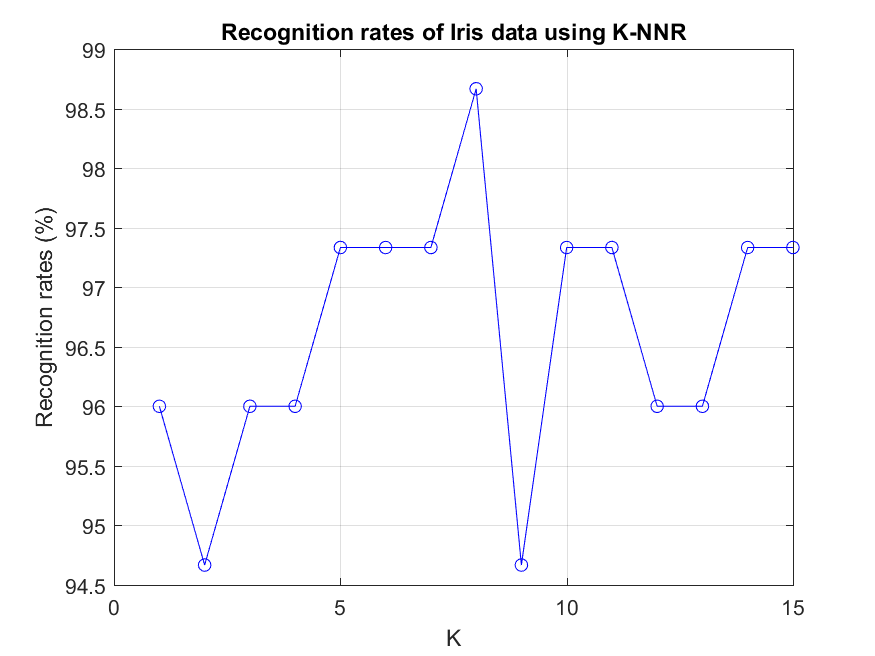It can be observed that a too small or too big value of K does not produce good performance. The best value of K, in this case, is 5 or 7 or 9, which all achieves an overall recognition rate of 98.67%. However, different applications require different values of K and usually we rely on exhaustive search as shown in this example to determine the best value of K.

To avoid a tie in the voting process of KNNC, usually we have the following strategies:

• You should choose a K that will not give a tie. For instance, when the number of classes is 2, the value of K should be set to an odd number such that no tie happens.
• We can also use the distance as a weighting factor for the voting. This is referred to as the distance-weighted KNNC, which usually gives a more robust result.

Data Clustering and Pattern Recognition (資料分群與樣式辨認)